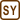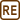## Two Connected Vessels

### Task number: 1799

Vessel A containing an ideal gas at 300 K and 5.0·105 Pa pressure is connected with a vessel B by a narrow tube. Vessel B has four times greater inner volume and contains the same gas heated until its temperature is 400 K and a pressure is 1.0·105 Pa. How high will be the resultant pressure of the entire system if we simultaneously turn a tube tap on and keep both vessels at their initial temperatures?

• #### Hint

Using the ideal gas law, we are able to determine the amount of gas in both vessels.

After connecting the vessels together, a part of the gas will move from the vessel A to the vessel B, so that both vessels would be at the same pressure. Pressure in each vessel again can be expressed by the ideal gas law. Furthermore, it suffices to note that the sum of the amounts of gas in each vessel separately before and after turning the tap on is the same.

• #### Notation

 TA = 300 K temperature of the vessel A pA = 5.0·105 Pa gas pressure in the vessel A VB = 4VA relation between the volumes of the two vessels TB = 400 K temperature of the vessel B pB = 1.0·105 Pa gas pressure in the vessel B p = ? resultant gas pressure
• #### Analysis

First, from the ideal gas law we express the amount of gas in both vessels before turning the connecting tap on.

After opening the tap in the connecting tube the gas in both vessels redistributes, so that both vessels are at the same pressure. To the gas in both vessels The ideal gas law can still be applied to the gas in both vessels, so we can use it to express the amount of gas in the vessels after turning the tap on.

Due to the fact that the gas was not leaking out into the surroundings, the total amount of gas in both vessels before and after turning on the tap must be the same. From there we express the required resultant pressure.

• #### Solution

The ideal gas law can be applied to the gas in vessel A in the form:

$p_AV_A\,=\,n_ART_A\,,$

where nA is the unknown chemical amount of gas in the vessel A, which we can express from this equation:

$n_A\,=\,\frac{p_AV_A}{RT_A}\,.$

Similarly, we express the chemical amount of gas in the vessel B:

$p_BV_B\,=\,n_BRT_B\hspace{15px}\Rightarrow\hspace{15px}n_B\,=\,\frac{p_BV_B}{RT_B}\,=\,\frac{4p_BV_A}{RT_B}\,.$

The chemical amount of gas in the vessel A after turning the tube tap on we denote as n1, and in vessel B – n2. Both of the amounts we also express from the ideal gas law and substitute the pressure p, which is the same in each vessel:

$pV_A\,=\,n_1RT_A\,\hspace{15px}\Rightarrow\hspace{15px}n_1\,=\,\frac{pV_A}{RT_A}$ $p4V_A\,=\,n_2RT_B\,\hspace{15px}\Rightarrow\hspace{15px}n_2\,=\,\frac{4pV_A}{RT_B}\,.$

Total chemical amount of gas in both vessels before turning the tap on and after that is the same, therefore it must hold that:

$n_A\,+\,n_B\,=\,n_1\,+\,n_2$ $\frac{p_AV_A}{RT_A}\,+\,\frac{4p_BV_A}{RT_B}\,=\,\frac{pV_A}{RT_A}\,+\,\frac{4pV_A}{RT_B}\,.$

We rearrange the last equation and express the required pressure p:

$\frac{V_A}{R}\left(\frac{p_A}{T_A}\,+\,\frac{4p_B}{T_B}\right)\,=\,\frac{pV_A}{R}\left(\frac{1}{T_A}\,+\,\frac{4}{T_B}\right)$ $p\,=\,\frac{p_AT_B+4p_BT_A}{T_AT_B}\frac{T_AT_B}{T_B+4T_A}\,=\,\frac{p_AT_B+4p_BT_A}{T_B+4T_A}\,.$

Substituting given values, we get:

$p\,=\,\frac{5\cdot{10^5}\cdot{400}\,+\,4\cdot{1}\cdot 10^5\cdot{300}}{400\,+\,4\cdot{300}}\,\mathrm{Pa}\,\dot{=}\,2{.}0\cdot{10^5}\,\mathrm{Pa}\,.$
• #### Answer

After turning on the tap in the connecting tube the gas pressure in both vessels will be about 2.0·105 Pa.